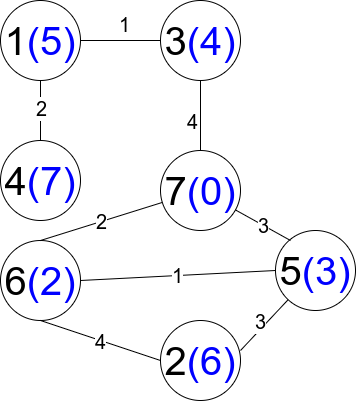Formatted question description: https://leetcode.ca/all/1786.html

# 1786. Number of Restricted Paths From First to Last Node

Medium

## Description

There is an undirected weighted connected graph. You are given a positive integer n which denotes that the graph has n nodes labeled from 1 to n, and an array edges where each edges[i] = [u_i, v_i, weight_i] denotes that there is an edge between nodes u_i and v_i with weight equal to weight_i.

A path from node start to node end is a sequence of nodes [z_0, z_1, z_2, ..., z_k] such that z_0 = start and z_k = end and there is an edge between z_i and z_(i+1) where 0 <= i <= k-1.

The distance of a path is the sum of the weights on the edges of the path. Let distanceToLastNode(x) denote the shortest distance of a path between node n and node x. A restricted path is a path that also satisfies that distanceToLastNode(z_i) > distanceToLastNode(z_(i+1)) where 0 <= i <= k-1.

Return the number of restricted paths from node 1 to node n. Since that number may be too large, return it modulo 10^9 + 7.

Example 1:Input: n = 5, edges = [[1,2,3],[1,3,3],[2,3,1],[1,4,2],[5,2,2],[3,5,1],[5,4,10]]

Output: 3

Explanation: Each circle contains the node number in black and its distanceToLastNode value in blue. The three restricted paths are:

1) 1 –> 2 –> 5

2) 1 –> 2 –> 3 –> 5

3) 1 –> 3 –> 5

Example 2:Input: n = 7, edges = [[1,3,1],[4,1,2],[7,3,4],[2,5,3],[5,6,1],[6,7,2],[7,5,3],[2,6,4]]

Output: 1

Explanation: Each circle contains the node number in black and its distanceToLastNode value in blue. The only restricted path is 1 –> 3 –> 7.

Constraints:

• 1 <= n <= 2 * 10^4
• n - 1 <= edges.length <= 4 * 10^4
• edges[i].length == 3
• 1 <= u_i, v_i <= n
• u_i != v_i
• 1 <= weight_i <= 10^5
• There is at most one edge between any two nodes.
• There is at least one path between any two nodes.

## Solution

Use a hash map to store each node’s adjacent nodes and the corresponding weights. Then use Dijkstra’s algorithm to find the shortest path from node n to each node. Finally, use dynamic programming to find the number of restricted paths that start from node 1 and end at node n.

class Solution {
static final int MODULO = 1000000007;
int restrictedPaths = 0;
int n = 0;
int[] dp;

public int countRestrictedPaths(int n, int[][] edges) {
this.n = n;
Map<Integer, List<int[]>> map = new HashMap<Integer, List<int[]>>();
for (int[] edge : edges) {
int u = edge, v = edge, weight = edge;
List<int[]> list1 = map.getOrDefault(u, new ArrayList<int[]>());
List<int[]> list2 = map.getOrDefault(v, new ArrayList<int[]>());
map.put(u, list1);
map.put(v, list2);
}
int[] distances = getShortestDistances(n, map);
dp = new int[n + 1];
dp[n] = 1;
return backtrack(distances, map, 1);
}

public int[] getShortestDistances(int n, Map<Integer, List<int[]>> map) {
int[] distances = new int[n + 1];
Arrays.fill(distances, Integer.MAX_VALUE);
distances[n] = 0;
PriorityQueue<Integer> priorityQueue = new PriorityQueue<Integer>(new Comparator<Integer>() {
public int compare(Integer node1, Integer node2) {
return distances[node1] - distances[node2];
}
});
priorityQueue.offer(n);
while (!priorityQueue.isEmpty()) {
int node = priorityQueue.poll();
int distance = distances[node];
List<int[]> list = map.getOrDefault(node, new ArrayList<int[]>());
for (int[] next : list) {
int nextNode = next, weight = next;
if (distance + weight < distances[nextNode]) {
distances[nextNode] = distance + weight;
priorityQueue.offer(nextNode);
}
}
}
return distances;
}

public int backtrack(int[] distances, Map<Integer, List<int[]>> map, int node) {
if (dp[node] == 0) {
int curDistance = distances[node];
List<int[]> list = map.getOrDefault(node, new ArrayList<int[]>());
for (int[] next : list) {
int nextNode = next;
if (distances[nextNode] < curDistance) {
int nextCount = backtrack(distances, map, nextNode);
dp[node] = (dp[node] + nextCount) % MODULO;
}
}
}
return dp[node];
}
}